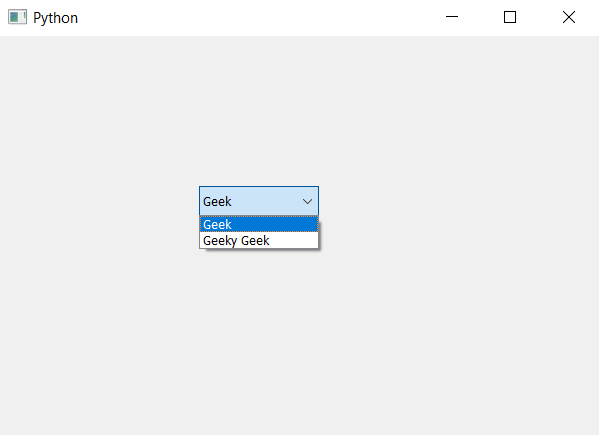# PyQt5 – Setting limit to number of items in ComboBox

• Last Updated : 22 Apr, 2020

In this article we will see how we can set limit to the number of items in the combo box. When we create a combo box there is no limit set to the items we can add any number of items although sometimes condition arises to set the maximum limit to the items.

In order to set the maximum limit to the number of items we use `setMaxCount` method.

Syntax : combo_box.setMaxCount(n)

Argument : It takes integer as argument

Action performed : This will set maximum limit to the number of items in combo box

Below is the implementation –

 `# importing libraries ` `from` `PyQt5.QtWidgets ``import` `*`  `from` `PyQt5 ``import` `QtCore, QtGui ` `from` `PyQt5.QtGui ``import` `*`  `from` `PyQt5.QtCore ``import` `*`  `import` `sys ` ` `  ` `  `class` `Window(QMainWindow): ` ` `  `    ``def` `__init__(``self``): ` `        ``super``().__init__() ` ` `  `        ``# setting title ` `        ``self``.setWindowTitle(``"Python "``) ` ` `  `        ``# setting geometry ` `        ``self``.setGeometry(``100``, ``100``, ``600``, ``400``) ` ` `  `        ``# calling method ` `        ``self``.UiComponents() ` ` `  `        ``# showing all the widgets ` `        ``self``.show() ` ` `  `    ``# method for widgets ` `    ``def` `UiComponents(``self``): ` ` `  `        ``# creating a combo box widget ` `        ``self``.combo_box ``=` `QComboBox(``self``) ` ` `  `        ``# setting geometry of combo box ` `        ``self``.combo_box.setGeometry(``200``, ``150``, ``120``, ``30``) ` ` `  `        ``# geek list ` `        ``geek_list ``=` `[``"Geek"``, ``"Geeky Geek"``, ``"Legend Geek"``, ``"Ultra Legend Geek"``] ` ` `  `        ``# adding list of items to combo box ` `        ``self``.combo_box.addItems(geek_list) ` ` `  `        ``# setting maximum number limit of items ` `        ``self``.combo_box.setMaxCount(``2``) ` ` `  `# create pyqt5 app ` `App ``=` `QApplication(sys.argv) ` ` `  `# create the instance of our Window ` `window ``=` `Window() ` ` `  `# start the app ` `sys.exit(App.``exec``()) `

Output :My Personal Notes arrow_drop_up
Recommended Articles
Page :